Advanced Database Management System - Tutorials and Notes: Minimum Edit Distance with Alignment

## Search Engine

Please visit, subscribe and share 10 Minutes Lectures in Computer Science

# How to use minimum edit distance to find the distance between two strings with the corresponding alignment using Backtrace?

## Minimum Edit Distance with Backtrace for Alignment

Minimum edit distance is the minimum number of editing operations (insertion, deletion, substitution) required to convert one string into another. Dynamic programming is the method used to find the minimum edit distance.
In the previous posts, we have discussed about the minimum edit distance. Either the basic version or the Levenshtein distance version is useful to find the distance. But the major question is how to align two strings after finding the distance. For example, if I am about to convert RIVER to SHIVER, I should conclude on the operations that I have to apply on each character of RIVER. To decide this, we need a corresponding alignment as per the minimum cost calculated. We can achieve this by using backtrace pointers in each cell. That is, while we are calculating the minimum cost for each cell (each sub-problem), we also include a pointer in that cell to remember how did we arrive at the cost for that particular cell. This pointer points to the previous cell which was used in the calculation of the cost.

In the example given below, we find the distance between the words DOG and COW using the basic Minimum Edit Distance algorithm. Also, the Step-by-step process is shown along with how to use backtrace pointers.

Step 1: Draw the edit distance matrix. In this, each word is preceded by # symbol which marks the empty string.
 # C O W # D O G

Step 2: Find the edit-distance values using minimum edit distance algorithm to convert # (row side) to #COW (column side) and populate appropriate cells with the calculated distance.
Number of operations required to convert;
·        # to # is 0.
·        # to #C is 1. That is, one insertion (no change with #, insert C)
·        # to #CO is 2. That is, two insertions (no change with #, insert C and O)
·        # to #COW is 3. That is, three insertions (no change with #, insert C, O and W)
 # C O W # 0 1 2 3 D O G

Step 3: Find the edit-distance values using minimum edit distance algorithm to convert # (column side) to #DOG (row side) and populate appropriate cells with the calculated distance.
Number of operations required to convert
·        # to # is 0.
·        # to #D is 1. [one insertion (no change with #, insert D]
·        # to #DO is 2. [two insertions (no change #, insert D and O]
·        # to #DOG is 3. [three insertions (no change #, insert D, O and G]
 # C O W # 0 1 2 3 D 1 O 2 G 3

So far, we have found the minimum edit distance for 7 sub-problems.
[# - # = 0, # - #C = 1, # - #CO = 2, # - #COW = 3, # - #D = 1, # - #DO = 2, and # - #DOG = 3]

Step 4: From this step onwards, we try to find the cost for a sub-problem by finding the minimum cost of three sub-problems and add 1 with that if the characters intersect at that cell are different. If the intersecting characters are same, then we add 0 with the diagonal cell value.
[Note: we have used A as the name for this matrix and included the index numbers for easy understanding]
Use the following table as a key to find the cost.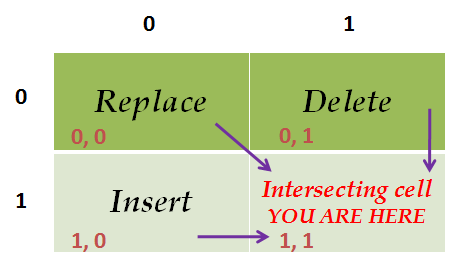If the row character ≠ column character,
The cost of the intersecting cell = min(replace, delete, insert).
If the row character = column character,
The cost of the intersecting cell = cost of the Replace cell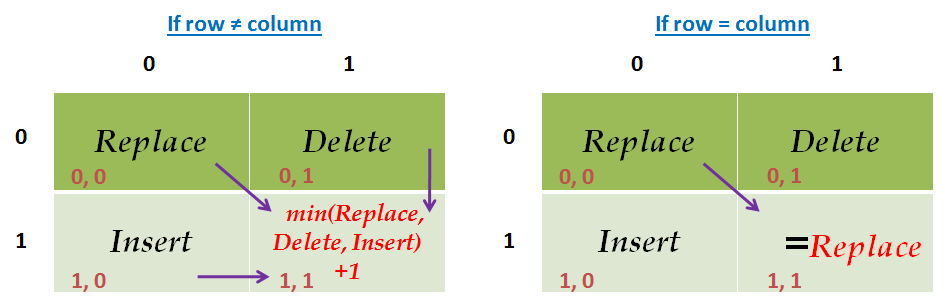Sub-problem: #D #C.
Intersecting cell: A[1, 1]
Same intersecting characters? NO.
Cost(A[1, 1])       = Min(A[0, 0], A[0, 1], A[1, 0]) + 1
= A[0, 0] + 1
= 0 + 1 = 1
As the cost derived from the cost of cell A[0, 0], a pointer is included from the current cell A[1, 1] to mark the back-trace path.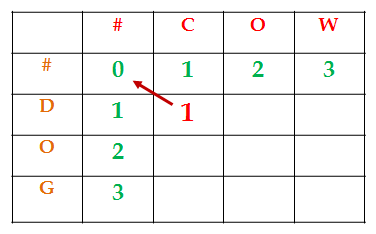Sub-problem: #D #CO.
Intersecting cell: A[1, 2]
Same intersecting characters? NO.
Cost(A[1, 2])       = Min(A[0, 1], A[0, 2], A[1, 1]) + 1
= A[1, 1] + 1
= 1 + 1 = 2
As the cost derived from the cost of cell A[1, 1], a pointer is included from the current cell A[1, 2] to mark the back-trace path.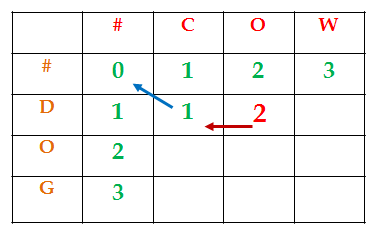Justification for choosing cell A[1, 1] as the previous cell: We have two cells (A[0,1] and A[1, 1]) with the same minimum cost 1. Which one to choose? We choose A[1, 1]. The reasons are;
We are trying to convert ‘D’ to ‘CO’ in this sub-problem
We already used the substitution operation (substitute character D with C).
So, the next character ‘O’ in the output can be derived with the insert operation only.
Hence, we have chosen the cell A[1, 1] which represents insert operation (refer the key table above) as the minimum valued cell

Sub-problem: #D #COW.
Intersecting cell: A[1, 3]
Same intersecting characters? NO.
Cost(A[1, 3])       = Min(A[0, 2], A[0, 3], A[1, 2]) + 1
= A[1, 2] + 1
= 2 + 1 = 3
As the cost derived from the cost of cell A[1, 2], a pointer is included from the current cell A[1, 3] to mark the back-trace path.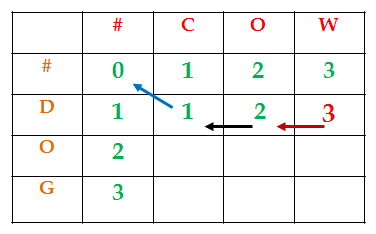Justification for choosing cell A[1, 2] as the previous cell: We have two cells (A[0, 2] and A[1, 2]) with the same minimum cost 1. Which one to choose? We choose A[1, 2]. The reasons are;
We are trying to convert ‘D’ to ‘COW’ in this sub-problem
We already used the substitution operation (substitute character D with C).
So, the next characters ‘O’ and ‘W’ in the output can be derived with the insert operation only.
Hence, we have chosen the cell A[1, 2] which represents insert operation (refer the key table above) as the minimum valued cell.

Sub-problem: #DO #C.
Intersecting cell: A[2, 1]
Same intersecting characters? NO.
Cost(A[2, 1])       = Min(A[1, 0], A[1, 1], A[2, 0]) + 1
= A[1, 1] + 1
= 1 + 1 = 2
As the cost derived from the cost of cell A[1, 1], a pointer is included from the current cell A[2, 1] to mark the back-trace path.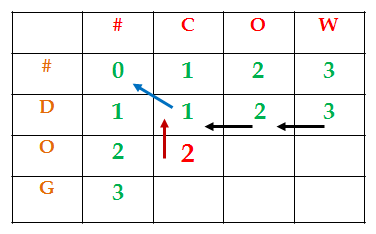Justification for choosing cell A[1, 1] as the previous cell: We have two cells (A[1, 0] and A[1, 1]) with the same minimum cost 1. Which one to choose? We choose A[1, 1]. The reasons are;
We are trying to convert ‘DO’ to ‘C’ in this sub-problem
We already used the substitution operation (substitute character D with C).
So, the next character ‘O’ has to be removed in the output. Hence, a delete operation used.
Hence, we have chosen the cell A[1, 1] which represents delete operation (refer the key table above) as the minimum valued cell.

Sub-problem: #DO #CO.
Intersecting cell: A[2, 2]
Same intersecting characters? YES
Cost(A[2, 2])       = Min(A[1, 1], A[1, 2], A[2, 1]) + 0
= A[1, 1] + 0
= 1 + 0 = 1
As the cost derived from the cost of cell A[1, 1], a pointer is included from the current cell A[2, 2] to mark the back-trace path.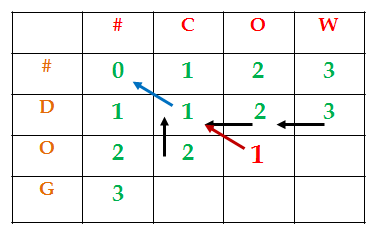Sub-problem: #DO #COW.
Intersecting cell: A[2, 3]
Same intersecting characters? NO.
Cost(A[2, 3])       = Min(A[1, 2], A[1, 3], A[2, 2]) + 1
= A[2, 2] + 1
= 1 + 1 = 2
As the cost derived from the cost of cell A[2, 2], a pointer is included from the current cell A[2, 3] to mark the back-trace path.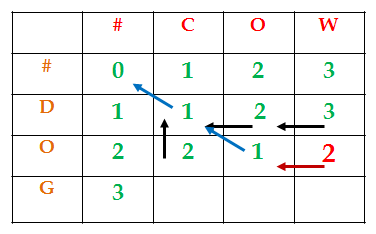Sub-problem: #DOG #C.
Intersecting cell: A[3, 1]
Same intersecting characters? NO.
Cost(A[3, 1])       = Min(A[2, 0], A[2, 1], A[3, 0]) + 1
= A[2, 1] + 1
= 2 + 1 = 3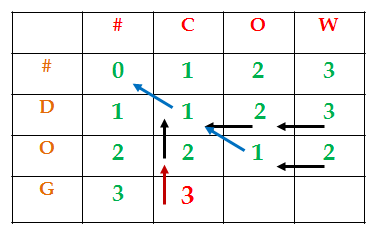Justification for choosing cell A[3, 1] as the previous cell: We have two cells (A[2, 0] and A[2, 1]) with the same minimum cost 1. Which one to choose? We choose A[2, 1]. The reasons are;
We are trying to convert ‘DOG’ to ‘C’ in this sub-problem
We already used the substitution operation (substitute character D with C) and delete operation (deleted a character ‘O’). [refer sub-problem #DO à #C]
So, the next character ‘G’ has to be removed in the output as well. Hence, a delete operation used.
Hence, we have chosen the cell A[2, 1] which represents delete operation (refer the key table above) as the minimum valued cell.

Sub-problem: #DOG #CO.
Intersecting cell: A[3, 2]
Same intersecting characters? NO
Cost(A[3, 2])       = Min(A[2, 1], A[2, 2], A[3, 1]) + 1
= A[2, 2] + 1
= 1 + 1 = 2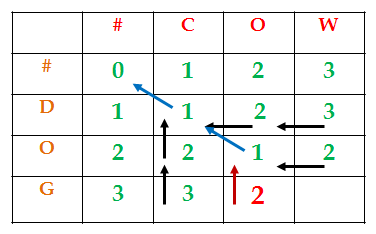Sub-problem: #DOG #COW.
Intersecting cell: A[3, 3]
Same intersecting characters? NO.
Cost(A[3, 3])       = Min(A[2, 2], A[2, 3], A[3, 2]) + 1
= A[2, 2] + 1
= 1 + 1 = 2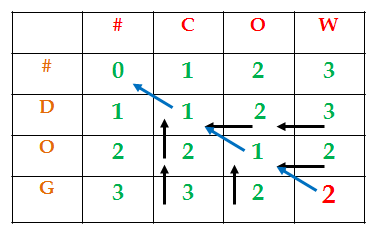Now, to learn the alignment, we need to traverse back from the last cell (the minimum edit distance between DOG and COW) using the pointer that starts at that cell. Refer the image below;
The cost at cell A[3, 3] was derived from cell A[2, 2] by incrementing the cost at A[2, 2] due to the substitution operation.
The cost at cell A[2, 2] was derived from cell A[1, 1]. The cost of A[1, 1] is used as it is because of No change. (the column character = row character in the intersecting cell).
The cost at cell A[1, 1] was derived from cell A[0, 0] by incrementing the cost at A[0, 0] due to the substitution operation.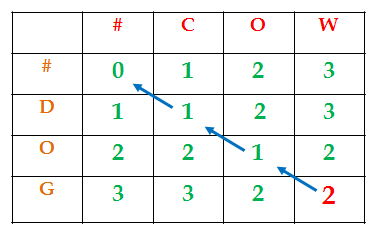Hence, the result of Minimum Edit Distance with alignment is;
 D O G | | | C O W Substitution No change Substitution

*************

Go to NLP Glossary